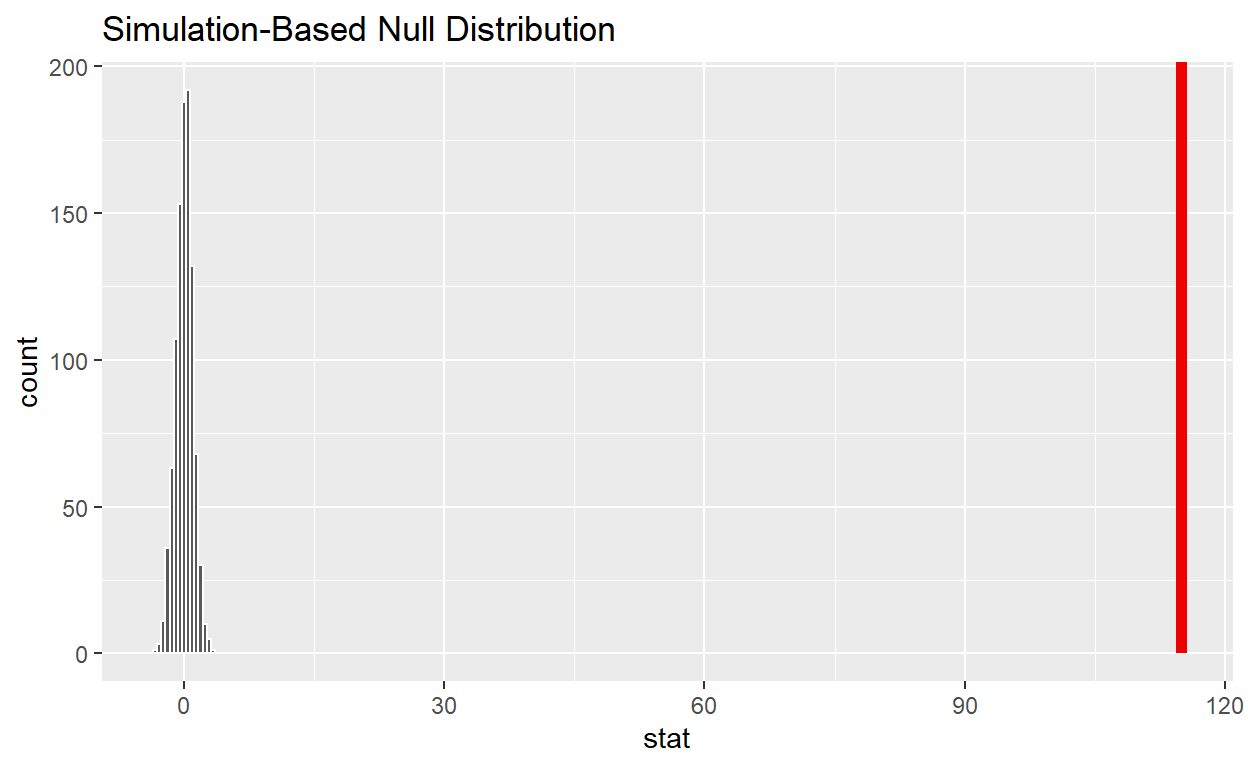# Hypothesis Testing

using computer simulation.Code for Quiz 13.

Contents

Question: t-test Q: is the mean number of hours per week 48?

Question: 2 sample t-test Q: Is the average number of hours worked the same for both genders?

Question: ANOVA Q: Is the average number of hours worked the same for all three status (fired, ok and promoted)?

Load the R packages we will use.

``````library(tidyverse)
library(infer)
library(skimr)
``````

#Question: t-test

• The data this quiz is a subset of `HR`
• Look at the variable definitions
• Note that the variables evaluation and salary have been recoded to be represented as words instead of numbers
• Set random seed generator to 123
``````set.seed(123)
``````

hr_1_tidy.csv is the name of your data subset

• Read into and assign to `hr`
``````hr  <- read_csv("https://estanny.com/static/week13/data/hr_1_tidy.csv", col_types = "fddfff")
``````

use the `skim` to summarize the data in `hr`

``````skim(hr)
``````
 Name hr Number of rows 500 Number of columns 6 _______________________ Column type frequency: factor 4 numeric 2 ________________________ Group variables None

Variable type: factor

skim_variable n_missing complete_rate ordered n_unique top_counts
gender 0 1 FALSE 2 fem: 260, mal: 240
evaluation 0 1 FALSE 4 bad: 153, fai: 142, goo: 106, ver: 99
salary 0 1 FALSE 6 lev: 93, lev: 92, lev: 91, lev: 84
status 0 1 FALSE 3 fir: 185, pro: 162, ok: 153

Variable type: numeric

skim_variable n_missing complete_rate mean sd p0 p25 p50 p75 p100 hist
age 0 1 40.60 11.58 20.2 30.37 41.00 50.82 59.9 ▇▇▇▇▇
hours 0 1 49.32 13.13 35.0 37.55 45.25 58.45 79.7 ▇▂▃▂▂

The mean hours worked per week is: 49.3

`specify` that `hours` is the variable of interest

``````hr  %>%
specify(response = hours)
``````
``````Response: hours (numeric)
# A tibble: 500 x 1
hours
<dbl>
1  36.5
2  55.8
3  35
4  52
5  35.1
6  36.3
7  40.1
8  42.7
9  66.6
10  35.5
# ... with 490 more rows``````

`hypothesize` that the average hours worked is 48

``````hr  %>%
specify(response = hours)  %>%
hypothesize(null = "point", mu = 48)
``````
``````Response: hours (numeric)
Null Hypothesis: point
# A tibble: 500 x 1
hours
<dbl>
1  36.5
2  55.8
3  35
4  52
5  35.1
6  36.3
7  40.1
8  42.7
9  66.6
10  35.5
# ... with 490 more rows``````

`generate` 1000 replicates representing the null hypothesis

``````hr  %>%
specify(response = hours)  %>%
hypothesise(null = "point", mu = 48)  %>%
generate(reps = 1000, type = "bootstrap")
``````
``````Response: hours (numeric)
Null Hypothesis: point
# A tibble: 500,000 x 2
# Groups:   replicate [1,000]
replicate hours
<int> <dbl>
1         1  33.7
2         1  34.9
3         1  46.6
4         1  33.8
5         1  61.2
6         1  34.7
7         1  37.9
8         1  39.0
9         1  62.8
10         1  50.9
# ... with 499,990 more rows``````

`calculate` the distribution of statistics from the generated data

• Assign the output `null_t_distribution`

• Display `null_t_distribution`

``````null_t_distribution  <- hr %>%
specify(response = age)  %>%
hypothesise(null = "point", mu = 48)  %>%
generate(reps = 1000, type = "bootstrap")  %>%
calculate(stat = "t")

null_t_distribution
``````
``````# A tibble: 1,000 x 2
replicate   stat
*     <int>  <dbl>
1         1  0.802
2         2 -0.706
3         3  1.33
4         4 -0.245
5         5 -1.11
6         6  0.382
7         7 -0.904
8         8  0.816
9         9  0.968
10        10  0.979
# ... with 990 more rows``````

`visualize` the simulated null distribution

``````visualise(null_t_distribution)
``````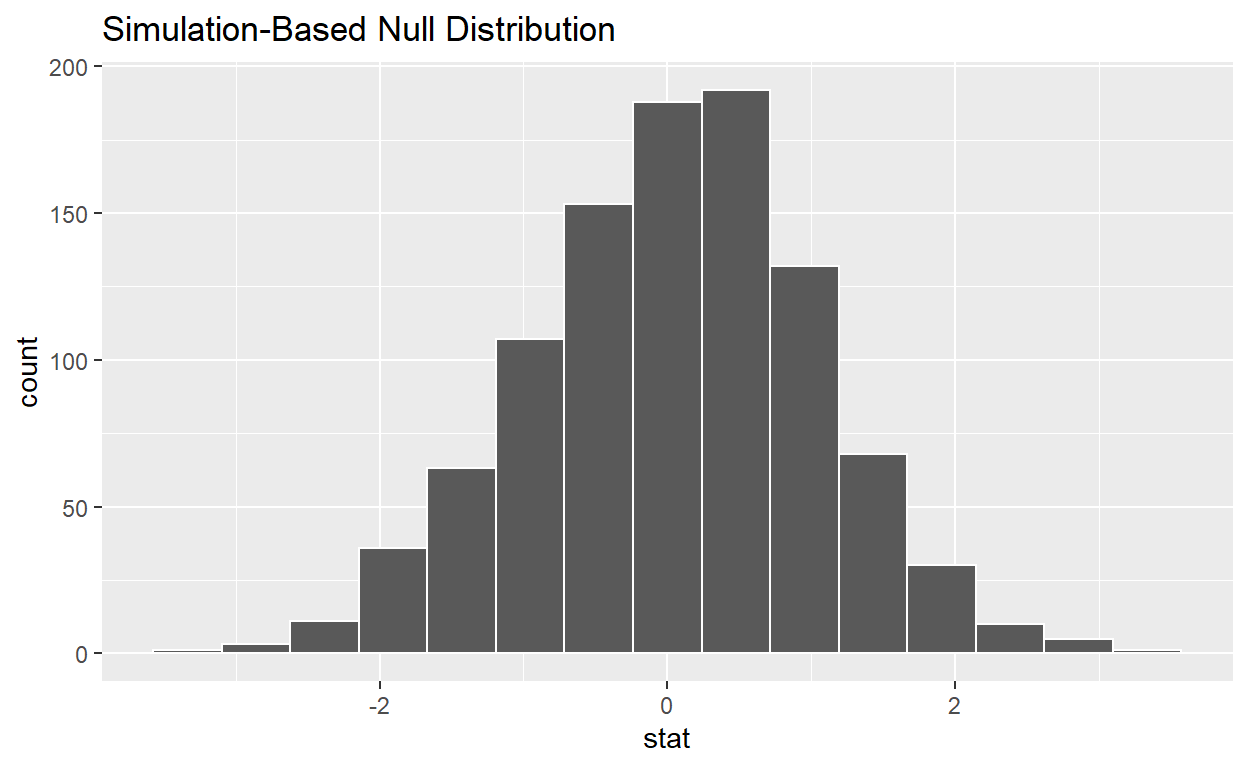`calculate` the statistic from your observed data - Assign the output `observed_t_statistic` - Display `observed_t_statistic`

``````observed_t_statistic  <- hr  %>%
specify(response = hours)  %>%
hypothesize(null = "point", mu = 48)  %>%
calculate(stat = "t")

observed_t_statistic
``````
``````# A tibble: 1 x 1
stat
<dbl>
1  2.25``````

get_p_value from the simulated null distribution and the observed statistic

``````null_t_distribution  %>%
get_p_value(obs_stat = 2.25, direction = "two-sided")
``````
``````# A tibble: 1 x 1
p_value
<dbl>
1   0.022``````

`shade_p_value` on the simulated null distribution

``````null_t_distribution  %>%
visualise() +
shade_p_value(obs_stat = 2.25, direction = "two-sided")
``````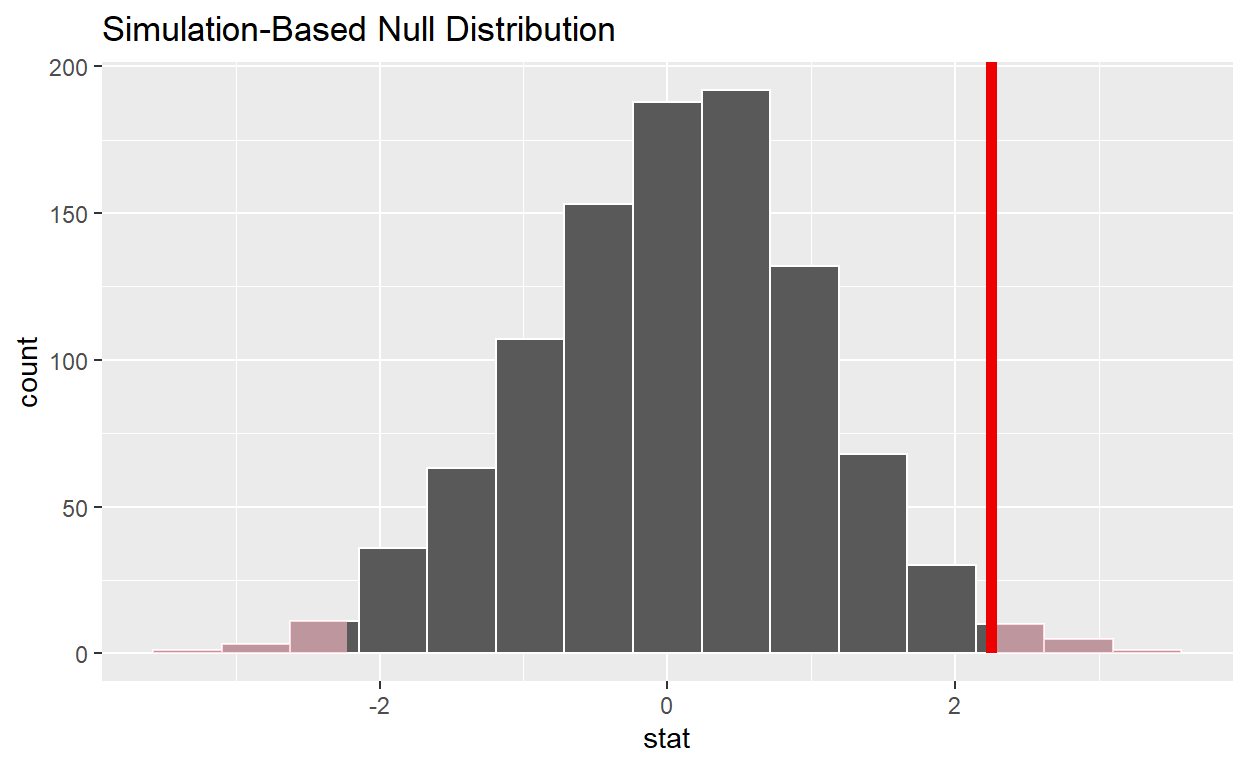#Question 2: sample t-test

hr_3_tidy.csv is the name of your data subset

• read it into and assign to `hr_2`
``````hr_2  <- read_csv("https://estanny.com/static/week13/data/hr_3_tidy.csv", col_types = "fddfff")
``````

#Q: Is the average number of hours worked the same for both genders in hr_2?

*use `skim` to summarize the data in `hr_2` by `gender`

``````hr_2 %>%
group_by(gender)  %>%
skim()
``````
 Name Piped data Number of rows 500 Number of columns 6 _______________________ Column type frequency: factor 3 numeric 2 ________________________ Group variables gender

Variable type: factor

skim_variable gender n_missing complete_rate ordered n_unique top_counts
evaluation male 0 1 FALSE 4 bad: 72, fai: 67, goo: 61, ver: 47
evaluation female 0 1 FALSE 4 bad: 76, fai: 71, goo: 61, ver: 45
salary male 0 1 FALSE 6 lev: 47, lev: 43, lev: 43, lev: 42
salary female 0 1 FALSE 6 lev: 51, lev: 46, lev: 45, lev: 43
status male 0 1 FALSE 3 fir: 98, pro: 81, ok: 68
status female 0 1 FALSE 3 fir: 98, pro: 91, ok: 64

Variable type: numeric

skim_variable gender n_missing complete_rate mean sd p0 p25 p50 p75 p100 hist
age male 0 1 38.23 10.86 20 28.9 37.9 47.05 59.9 ▇▇▇▇▅
age female 0 1 40.56 11.67 20 31.0 40.3 50.50 59.8 ▆▆▇▆▇
hours male 0 1 49.55 13.11 35 38.4 45.4 57.65 79.9 ▇▃▂▂▂
hours female 0 1 49.80 13.38 35 38.2 45.6 59.40 79.8 ▇▂▃▂▂

Use `geom_boxplot` to plot distributions of hours worked by gender

``````hr_2 %>%
ggplot(aes(x = gender, y = hours)) +
geom_boxplot()
``````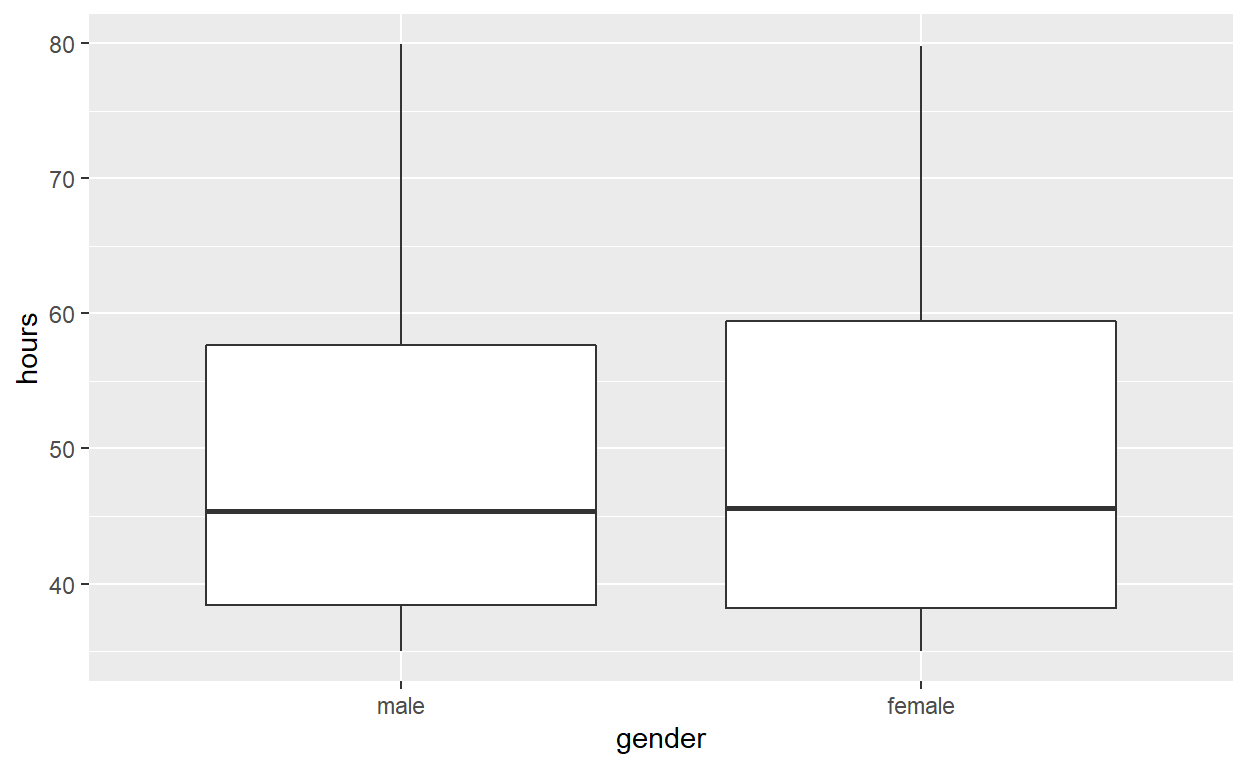`specify` the variables of interest are `hours` and `gender`

``````hr_2 %>%
specify(response = hours, explanatory = gender)
``````
``````Response: hours (numeric)
Explanatory: gender (factor)
# A tibble: 500 x 2
hours gender
<dbl> <fct>
1  49.6 male
2  39.2 female
3  63.2 female
4  42.2 male
5  54.7 male
6  54.3 female
7  37.3 female
8  45.6 female
9  35.1 female
10  53   male
# ... with 490 more rows``````

`hypothesize` that the number of hours worked and gender are independent

``````hr_2 %>%
specify(response = hours, explanatory = gender)  %>%
hypothesise(null = "independence")
``````
``````Response: hours (numeric)
Explanatory: gender (factor)
Null Hypothesis: independence
# A tibble: 500 x 2
hours gender
<dbl> <fct>
1  49.6 male
2  39.2 female
3  63.2 female
4  42.2 male
5  54.7 male
6  54.3 female
7  37.3 female
8  45.6 female
9  35.1 female
10  53   male
# ... with 490 more rows``````

`generate` 1000 replicates representing the null hypothesis

``````hr_2 %>%
specify(response = hours, explanatory = gender)  %>%
hypothesize(null = "independence")  %>%
generate(reps = 1000, type = "permute")
``````
``````Response: hours (numeric)
Explanatory: gender (factor)
Null Hypothesis: independence
# A tibble: 500,000 x 3
# Groups:   replicate [1,000]
hours gender replicate
<dbl> <fct>      <int>
1  55.7 male           1
2  35.5 female         1
3  35.1 female         1
4  44.2 male           1
5  52.8 male           1
6  46   female         1
7  41.2 female         1
8  52.9 female         1
9  35.6 female         1
10  35   male           1
# ... with 499,990 more rows``````

`calculate` the distribution of statistics from the generated data

• Assign the output `null_distribution_2_sample_permute`
• Display `null_distribution_2_sample_permute`
``````null_distribution_2_sample_permute  <- hr_2 %>%
specify(response = hours, explanatory = gender)  %>%
hypothesize(null = "independence")  %>%
generate(reps = 1000, type = "permute")  %>%
calculate(stat = "t", order = c("female", "male"))

null_distribution_2_sample_permute
``````
``````# A tibble: 1,000 x 2
replicate    stat
*     <int>   <dbl>
1         1 -1.81
2         2 -1.29
3         3  0.0525
4         4 -0.793
5         5  0.826
6         6  0.429
7         7  0.0843
8         8 -0.264
9         9  2.42
10        10  0.603
# ... with 990 more rows``````

`visualize` the simulated null distribution

``````visualise(null_distribution_2_sample_permute)
``````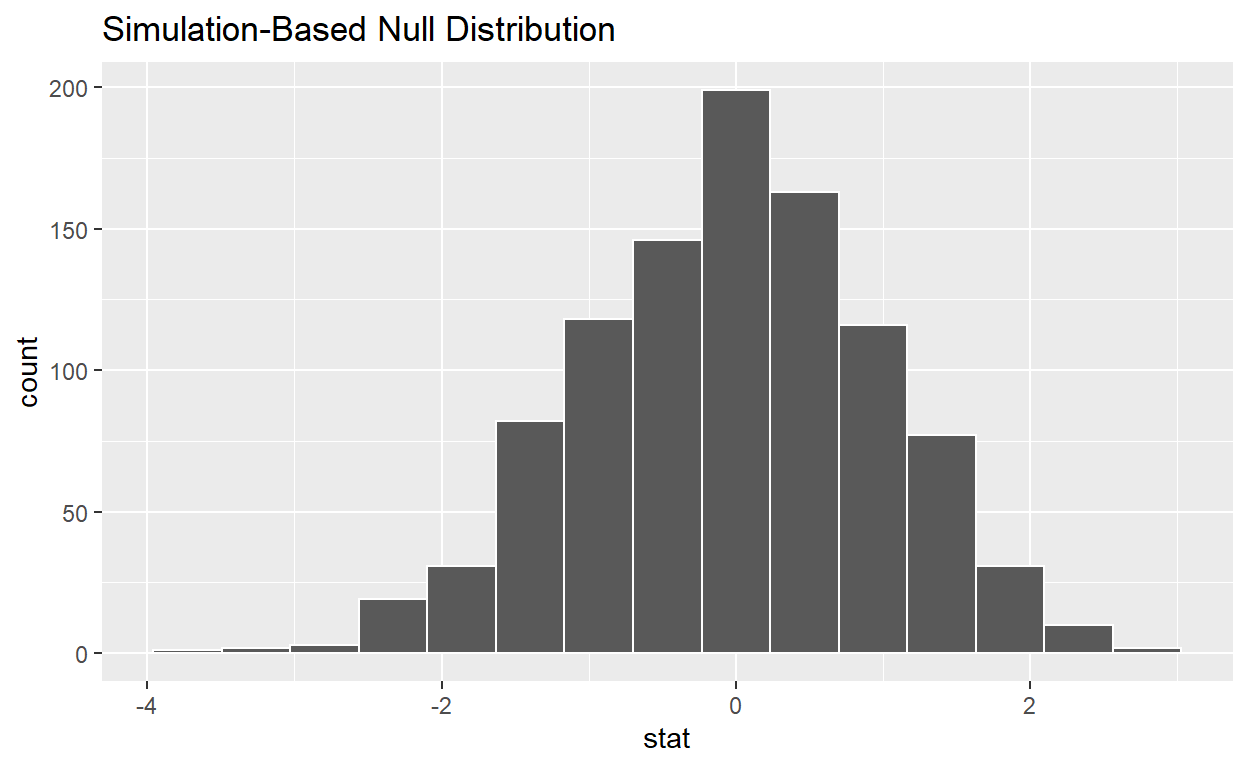`calculate` the statistic from your observed data

• Assign the output `observed_t_2_sample_stat`
• Display `observed_t_2_sample_stat`
``````observed_t_2_sample_stat  <- hr_2 %>%
specify(response = hours, explanatory = gender)  %>%
calculate(stat = "t", order = c("female", "male"))

observed_t_2_sample_stat
``````
``````# A tibble: 1 x 1
stat
<dbl>
1 0.208``````

get_p_value from the simulated null distribution and the observed statistic

``````null_t_distribution  %>%
get_p_value(obs_stat = 0.208, direction = "two-sided")
``````
``````# A tibble: 1 x 1
p_value
<dbl>
1   0.892``````

`shade_p_value` on the simulated null distribution

``````null_t_distribution  %>%
visualise() +
shade_p_value(obs_stat = 0.208, direction = "two-sided")
``````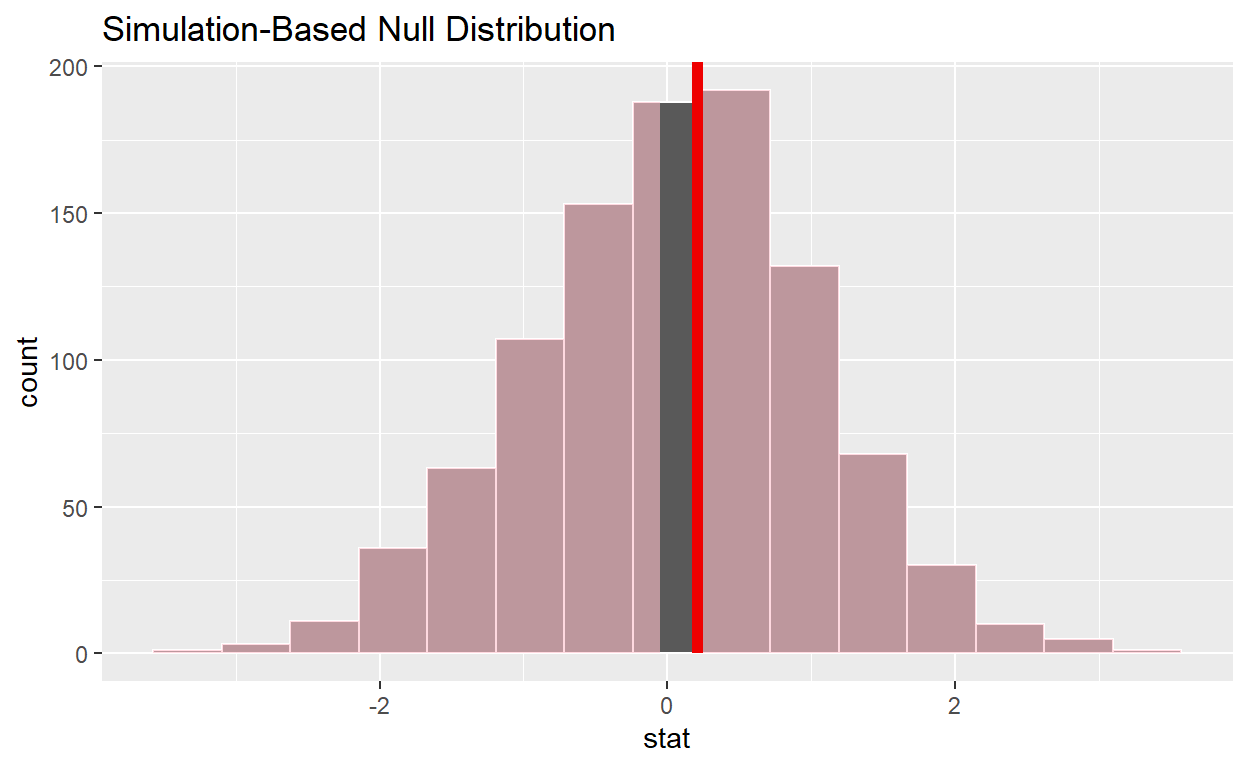#Question: ANOVA

*`hr_1_tidy.csv` is the name of your data subset

• read it into and assign to `hr_anova`
``````hr_anova  <- read_csv("https://estanny.com/static/week13/data/hr_1_tidy.csv", col_types = "fddfff")
``````

#Q: is the average number of hours worked the same for all three status (fired, ok, and promoted)?

use `skim` to summarize the data in `hr_anova` by `status`

``````hr_anova %>%
group_by(status)  %>%
skim()
``````
 Name Piped data Number of rows 500 Number of columns 6 _______________________ Column type frequency: factor 3 numeric 2 ________________________ Group variables status

Variable type: factor

skim_variable status n_missing complete_rate ordered n_unique top_counts
gender fired 0 1 FALSE 2 fem: 96, mal: 89
gender ok 0 1 FALSE 2 fem: 77, mal: 76
gender promoted 0 1 FALSE 2 fem: 87, mal: 75
evaluation fired 0 1 FALSE 4 bad: 65, fai: 63, goo: 31, ver: 26
evaluation ok 0 1 FALSE 4 bad: 69, fai: 59, goo: 15, ver: 10
evaluation promoted 0 1 FALSE 4 ver: 63, goo: 60, fai: 20, bad: 19
salary fired 0 1 FALSE 6 lev: 41, lev: 37, lev: 32, lev: 32
salary ok 0 1 FALSE 6 lev: 40, lev: 37, lev: 29, lev: 23
salary promoted 0 1 FALSE 6 lev: 37, lev: 35, lev: 29, lev: 23

Variable type: numeric

skim_variable status n_missing complete_rate mean sd p0 p25 p50 p75 p100 hist
age fired 0 1 38.64 11.43 20.2 28.30 38.30 47.60 59.6 ▇▇▇▅▆
age ok 0 1 41.34 12.11 20.3 31.00 42.10 51.70 59.9 ▆▆▆▆▇
age promoted 0 1 42.13 10.98 21.0 33.40 42.95 50.98 59.9 ▆▅▆▇▇
hours fired 0 1 41.67 7.88 35.0 36.10 38.90 43.90 75.5 ▇▂▁▁▁
hours ok 0 1 48.05 11.65 35.0 37.70 45.60 56.10 78.2 ▇▃▃▂▁
hours promoted 0 1 59.27 12.90 35.0 51.12 60.10 70.15 79.7 ▆▅▇▇▇
• Employees that were fired worked an average of 41.7 hours per week
• Employees that were ok worked an average of 48.0 hours per work
• Employees that were promoted worled an average of 59.3 hours per week

*Use `geom_boxplot` to plot distributions of hours worked by status

``````hr_anova %>%
ggplot(aes(x = status, y = hours)) +
geom_boxplot()
``````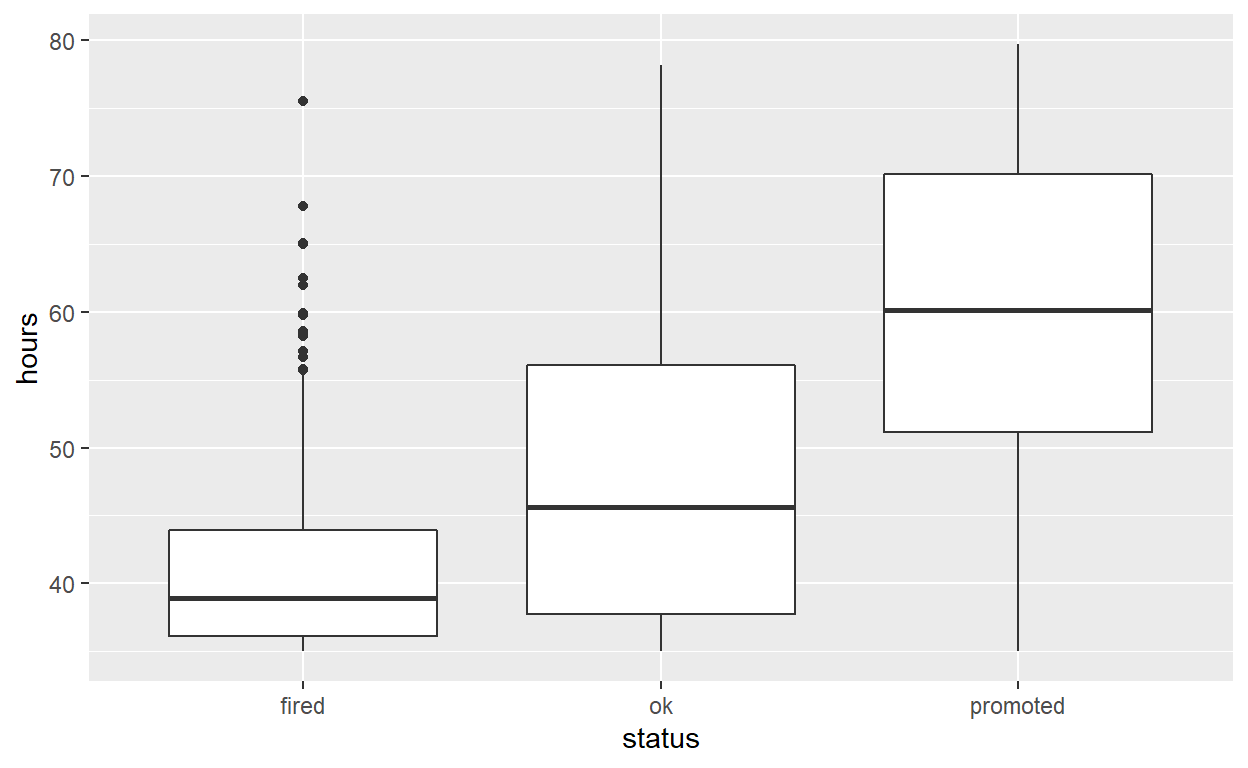*`specify` the variables of interest are `hours` and `status`

``````hr_anova %>%
specify(response = hours, explanatory = status)
``````
``````Response: hours (numeric)
Explanatory: status (factor)
# A tibble: 500 x 2
hours status
<dbl> <fct>
1  36.5 fired
2  55.8 ok
3  35   fired
4  52   promoted
5  35.1 ok
6  36.3 ok
7  40.1 promoted
8  42.7 fired
9  66.6 promoted
10  35.5 ok
# ... with 490 more rows``````

`hypothesize` that the number of hours worked and status are independent

``````hr_anova  %>%
specify(response = hours, explanatory = status)  %>%
hypothesise(null = "independence")
``````
``````Response: hours (numeric)
Explanatory: status (factor)
Null Hypothesis: independence
# A tibble: 500 x 2
hours status
<dbl> <fct>
1  36.5 fired
2  55.8 ok
3  35   fired
4  52   promoted
5  35.1 ok
6  36.3 ok
7  40.1 promoted
8  42.7 fired
9  66.6 promoted
10  35.5 ok
# ... with 490 more rows``````

`generate` 1000 replicates representing the null hypothesis

``````hr_anova %>%
specify(response = hours, explanatory = status)  %>%
hypothesise(null = "independence")  %>%
generate(reps = 1000, type = "permute")
``````
``````Response: hours (numeric)
Explanatory: status (factor)
Null Hypothesis: independence
# A tibble: 500,000 x 3
# Groups:   replicate [1,000]
hours status   replicate
<dbl> <fct>        <int>
1  40.3 fired            1
2  40.3 ok               1
3  37.3 fired            1
4  50.5 promoted         1
5  35.1 ok               1
6  67.8 ok               1
7  39.3 promoted         1
8  35.7 fired            1
9  40.2 promoted         1
10  38.4 ok               1
# ... with 499,990 more rows``````

`calculate` the distribution of statistics from the generated data

• Assign the output `null_distribution_anova`
• Display `null_distribution_anova`
``````null_distribution_anova  <- hr_anova %>%
specify(response = hours, explanatory = gender)  %>%
hypothesise(null = "independence")  %>%
generate(reps = 1000, type = "permute")  %>%
calculate(stat = "F")

null_distribution_anova
``````
``````# A tibble: 1,000 x 2
replicate   stat
*     <int>  <dbl>
1         1 0.365
2         2 0.650
3         3 0.185
4         4 0.0184
5         5 0.163
6         6 0.0194
7         7 4.92
8         8 2.11
9         9 0.341
10        10 0.855
# ... with 990 more rows``````

`visualize` the simulated null distribution

``````visualise(null_distribution_anova)
``````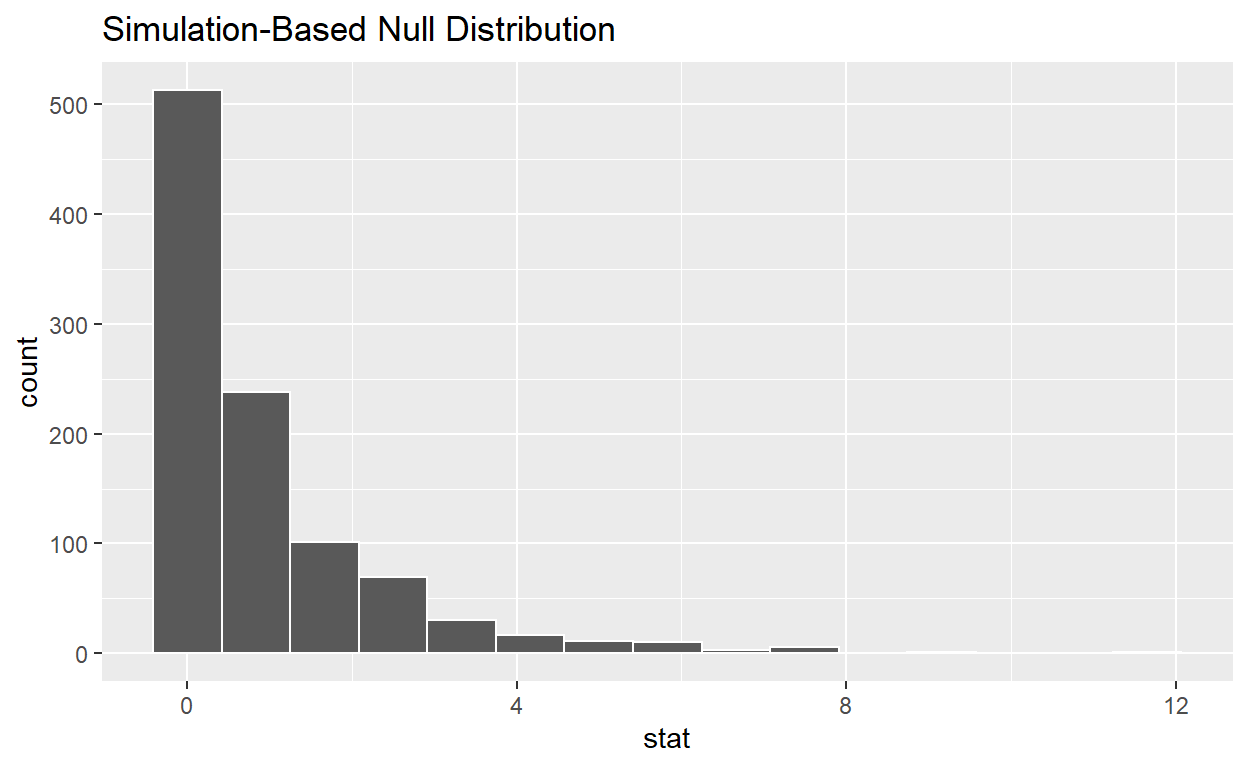`calculate` the statistic from your observed data - Assign the output `observed_f_sample_stat` - Display `observed_f_sample_stat`

``````observed_f_sample_stat  <- hr_anova %>%
specify(response = hours, explanatory = status)  %>%
calculate(stat = "F")

observed_f_sample_stat
``````
``````# A tibble: 1 x 1
stat
<dbl>
1  115.``````

get_p_value from the simulated null distribution

``````null_distribution_anova  %>%
get_p_value(obs_stat = 115, direction = "greater")
``````
``````# A tibble: 1 x 1
p_value
<dbl>
1       0``````

`shade_p_value` on the simulated null distribution

``````null_t_distribution  %>%
visualise() +
shade_p_value(obs_stat = 115, direction = "greater")
``````